Coordinates and Axes

In order to set up the framework that will enable us to deal easily with objects in higher-dimensional space, we develop briefly some of the structures we can use to locate numbers, number pairs, and number triples, in coordinate geometries of one, two, and three dimensions.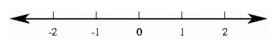Coordinates on the number line.

On a line, we may choose an origin, labeled 0, and a unit point, labeled 1. The origin sets a starting point, and the distance between the origin and the unit point establishes a scale. Any point on the ray from 0 through 1 is specified by its distance from the origin, represented as a multiple of the distance from 0 to 1. Points on the oppositely directed ray are also identified by their distance, but in this case the number is preceded by a negative sign. In this way, each point corresponds to a real number, its coordinate, and each real number corresponds to a single point on the line. The line is a one-dimensional space.

The plane is a two-dimensional space. In order to set up coordinates for points of the plane, we start with two number lines, called coordinate axes, intersecting at their common origin, labeled (0, 0). We label points on the first axis (x, 0) where x is the coordinate on the first number line, and points on the second axis as (0, y). Through any point in the plane we may draw lines parallel to the coordinate axes, meeting the first axis in a point labeled (x, 0) and the second in a point labeled (0, y). That point's location with respect to this choice of coordinate axes is then completely determined by the number pair (x, y). The point (x, y) is the fourth vertex of a parallelogram with one vertex at the origin and the other vertices at (x, 0) and (0, y). In coordinate geometry, we express this geometric construction by defining the coordinates of the sum of two point pairs as the sum of the corresponding coordinates:

(a, c) + (b, d) = (a + b, c + d)

For an arbitrary point, we find (x, y) = (x, 0) + (0, y), so any point can be expressed as a sum of points on the coordinate axes. In this coordinate system, we can define the unit square with the four corner points labeled (0, 0), (1, 0), (1, 1), and (0, 1).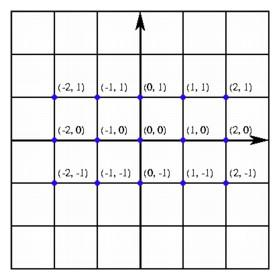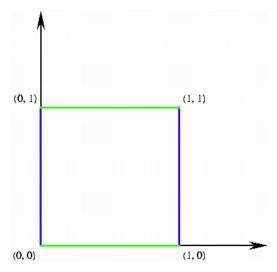Coordinates in the plane (left); the unit square in two-dimensional coordinate space (right).

In order to set up coordinates for points in ordinary three-dimensional space, we start with three number lines intersecting at their common origin (0, 0, 0). We label points on the first axis (x, 0, 0), points on the second axis (0, y, 0), and points on the third axis (0, 0, z). As in the case of the plane, we define the coordinates of the sum of two triples as the sum of the corresponding coordinates. The sums of points on the first two axes determine the 1-2-coordinate plane containing all points of the form (x, y, 0) = (x, 0, 0) + (0, y, 0). Similarly, the points on the 1-3-coordinate plane have coordinates (x, 0, z), and the points on the 2-3-coordinate plane are of the form (0, y, z). Through any point in three-space labeled (x, y, z), there are three planes parallel to these coordinate planes, meeting the first axis in the point (x, 0, 0), the second in the point (0, y, 0), and the third in a point (0, 0, z). Any point (x, y, z) can therefore be written as a sum of points on the parallelepiped with one vertex at the origin. In this coordinate system, we can define the unit cube with the eight corner points labeled (0, 0, 0), (1, 0, 0), (1, 1, 0), (0, 1, 0), (0, 0, 1), (1, 0, 1), (1, 1, 1), and (0, 1, 1).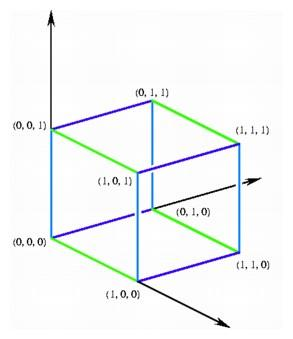The unit cube in three-dimensional coordinate space.

It is no accident that we have used the same basic language of axes and coordinates in describing the two-dimensional geometry of the plane and the three-dimensional geometry of ordinary space. Such a common description expresses a profound relationship between the two spaces. As we describe objects in the plane, we can often easily identify corresponding objects in space. And the analogy goes further -- we may use the same language to define a system of number quadruples, a four-dimensional hyperspace.

In the case of four-dimensional space, we can no longer base our concepts on familiar constructions of lines and planes. We begin with the coordinate representation itself, and ask what we can say about the space of all four-tuples of real numbers (x, y, u, v). Guided by our experience in the plane and in three-space, we designate the points of the form (x, 0, 0, 0) to be the first coordinate axis. Similarly, the points of the form (0, y, 0, 0) determine the second coordinate axis, while points of the form (0, 0, u, 0) compose the third axis, and those of the form (0, 0, 0, v) constitute the fourth coordinate axis. The point with label (0, 0, 0, 0), the intersection of these four axes, is the origin of the coordinate system. As in the case of the plane and three-space, we add two four-tuples by adding their corresponding coordinates. Thus every point in four-space can be expressed as the sum of four points lying on the coordinate axes.

Any pair of these coordinate axes determines a coordinate plane, for example the 2-3-coordinate plane containing all points of the form (0, y, u, 0). Any three of the four coordinate axes determine a coordinate hyperplane. Through any point in four-space there are four hyperplanes parallel to the coordinate hyperplanes, meeting the first axis in (x, 0, 0, 0), the second in (0, y, 0, 0), the third in (0, 0, u, 0), and the fourth in (0, 0, 0, v). With respect to this choice of coordinate axes, any point is completely determined by the number quadruple (x, y, u, v), and the point is the sixteenth vertex of a parallelotope with one vertex at the origin. In this way we obtain a coordinate system for four-dimensional space. In this coordinate system, we can define the unit hypercube with the 16 corner points labeled: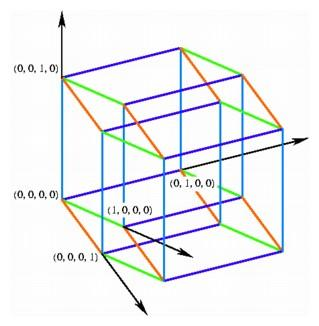The unit hypercube in four-dimensional coordinate space.

(0, 0, 0, 0), (1, 0, 0, 0), (1, 1, 0, 0), (0, 1, 0, 0),
(0, 0, 1, 0), (1, 0, 1, 0), (1, 1, 1, 0), (0, 1, 1, 0),
(0, 0, 1, 1), (1, 0, 1, 1), (1, 1, 1, 1), (0, 1, 1, 1),
(0, 0, 0, 1), (1, 0, 0, 1), (1, 1, 0, 1), (0, 1, 0, 1)

There is nothing to stop us from carrying out the same abstract description of a collection of five-tuples, or n-tuples in n-space. In once sense, we can say that n-dimensional space is exactly the collection of all n-tuples of real numbers, but this ignores the rich geometric content of n-dimensional geometry. We can gain additional insight into the relationships of number pairs, triples, or n-tuples by graphing them in lower-dimensional spaces, like the plane or three-space.